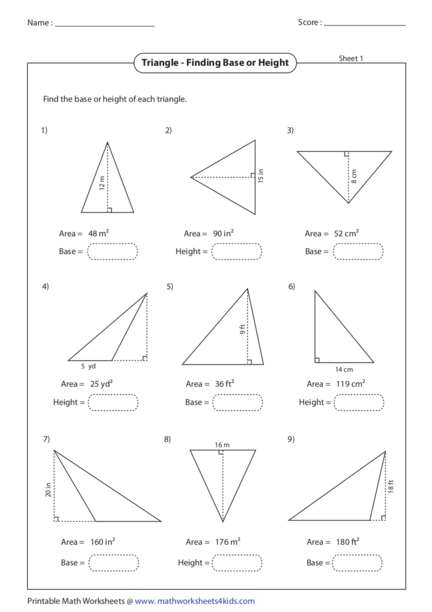# Finding Base or Height of triangleContributed by:This is a worksheet on finding the Base or Height of a triangle.
1. Name : Score :
Sheet 1
Triangle - Finding Base or Height
Find the base or height of each triangle.
1) 2) 3)
15 in
8 cm
12 m
Area = 48 m2 Area = 90 in2 Area = 52 cm2
Base = Height = Base =
4) 5) 6)
9 ft
5 yd 14 cm
Area = 25 yd2 Area = 36 ft2 Area = 119 cm2
Height = Base = Height =
7) 8) 9)
16 m
18 ft
20 in
Area = 160 in2 Area = 176 m2 Area = 180 ft2
Base = Height = Base =
Printable Math Worksheets @ www. mathworksheets4kids.com
2. Name : Score :
Sheet 1
Triangle - Finding Base or Height
Find the base or height of each triangle.
1) 2) 3)
15 in
8 cm
12 m
Area = 48 m2 Area = 90 in2 Area = 52 cm2
Base = 8m Height = 12 in Base = 13 cm
4) 5) 6)
9 ft
5 yd 14 cm
Area = 25 yd2 Area = 36 ft2 Area = 119 cm2
Height = 10 yd Base = 8 ft Height = 17 cm
7) 8) 9)
16 m
18 ft
20 in
Area = 160 in2 Area = 176 m2 Area = 180 ft2
Base = 16 in Height = 22 m Base = 20 ft
Printable Math Worksheets @ www. mathworksheets4kids.com
3. Name : Score :
Sheet 2
Triangle - Finding Base or Height
Find the base or height of each triangle.
1) 2) 3)
19 m
20 cm 16 ft
Area = 180 cm2 Area = 228 m2 Area = 152 ft2
Height = Base = Height =
4) 5) 6)
14 cm
16 in
6 yd
Area = 42 yd2 Area = 96 in2 Area = 112 cm2
Base = Base = Height =
7) 8) 9)
22 ft
15 m 8 in
Area = 150 m2 Area = 52 in2 Area = 165 ft2
Height = Height = Base =
Printable Math Worksheets @ www. mathworksheets4kids.com
4. Name : Score :
Sheet 2
Triangle - Finding Base or Height
Find the base or height of each triangle.
1) 2) 3)
19 m
20 cm 16 ft
Area = 180 cm2 Area = 228 m2 Area = 152 ft2
Height = 18 cm Base = 24 m Height = 19 ft
4) 5) 6)
14 cm
16 in
6 yd
Area = 42 yd2 Area = 96 in2 Area = 112 cm2
Base = 14 yd Base = 12 in Height = 16 cm
7) 8) 9)
22 ft
15 m 8 in
Area = 150 m2 Area = 52 in2 Area = 165 ft2
Height = 20 m Height = 13 in Base = 15 ft
Printable Math Worksheets @ www. mathworksheets4kids.com
5. Name : Score :
Sheet 3
Triangle - Finding Base or Height
Find the base or height of each triangle.
1) 2) 3)
14 cm
24 m 20 in
Area = 252 m2 Area = 133 cm2 Area = 170 in2
Height = Base = Height =
4) 5) 6)
8 ft
25 cm
4 yd
Area = 40 ft2 Area = 26 yd2 Area = 250 cm2
Base = Height = Base =
7) 8) 9)
21 in
16 ft
5m
Area = 20 m2 Area = 120 ft2 Area = 126 in2
Height = Base = Height =
Printable Math Worksheets @ www. mathworksheets4kids.com
6. Name : Score :
Sheet 3
Triangle - Finding Base or Height
Find the base or height of each triangle.
1) 2) 3)
14 cm
24 m 20 in
Area = 252 m2 Area = 133 cm2 Area = 170 in2
Height = 21 m Base = 19 cm Height = 17 in
4) 5) 6)
8 ft
25 cm
4 yd
Area = 40 ft2 Area = 26 yd2 Area = 250 cm2
Base = 10 ft Height = 13 yd Base = 20 cm
7) 8) 9)
21 in
16 ft
5m
Area = 20 m2 Area = 120 ft2 Area = 126 in2
Height = 8m Base = 15 ft Height = 12 in
Printable Math Worksheets @ www. mathworksheets4kids.com